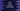# Dart program to round a number to its nearest value## Dart program to round a number to its nearest value:

In this dart programming example, we will learn how to round a number to its nearest value. Dart Number class provides round method that can be used to round a number.

### Syntax of Number.round():

round method is defined as below:

``Number.round()``

It returns an integer value. This is a value rounded the number to its closest integer.

### Example of Number.round():

Let’s take a look at the below example:

``````void main() {
var number_one = 10.6;
var number_two = -10.6;
var number_three = 11;
var number_four = -11.7;

print(number_one.round());
print(number_two.round());
print(number_three.round());
print(number_four.round());
}``````

If I run the above program, it will print the below output:

``````11
-11
11
-12``````